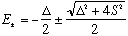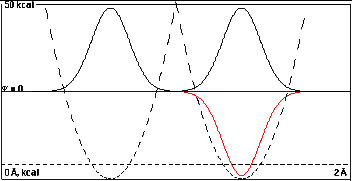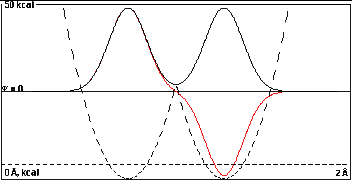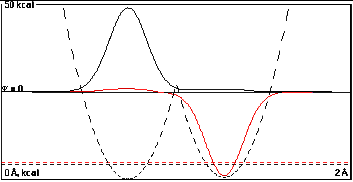Using Proton Tunnelling in a Double Minimum Potential to Understand
the Role of Overlap and Energy Match in Bonding and Antibonding MOs

Bonding and antibonding molecular orbitals (MOs) for electrons can be approximated as a sum and a difference, respectively, of atomic orbitals (AOs) centered on the two bonded atoms. How strongly bonding or antibonding the MOs are, that is how much they are shifted from the energies of the component AOs, depends on the overlap (S) and energy difference (D) of the component AOs.

Internalizing this idea is key to using MOs to understand chemical bonding.
 Some mathematically-minded individuals get the idea best by contemplating a formula that expresses the MO energies in terms of overlap and energy difference, S and D. Individuals who are attracted to physical chemistry are often of this type.Other less mathematically adroit individuals, including organic chemists like myself, are more comfortable if they have a clear graphical picture that explains the role of S and D. This page supplies a set of such pictures. If carefully considered, they yield good insight into the formation of bonding and antibonding MOs from AOs.

The approach used here is not to consider the three-dimensional electron in the double-well Coulombic potential of two nuclei but to consider the one-dimensional problem of a proton in a double-well harmonic potential. We have already treated this simpler problem quantum-mechanically using Erwin meets Goldilocks. The problems are very closely related, and show the same kind of response to S and D.

Technical details: The graphs were generated with mass = 1 amu ; double-well potential with no coupling and the two single-bond wells separated by 0.75, 0.55, 0.35, and 0.15 Å. In the first series of four plots the wells are degenerate, D = 0. In the second series the well on the right is raised by 0.5 kcal/mole. In all cases the width of the plot is 2 Å, and the dashed potential energy curve refers to an ordinate that goes from 0 kcal/mole at the bottom to 50 kcal/mole at the top. As usual, the horizontal dashed lines show the total energy for the two lowest energy solutions of this Schroedinger equation.
I. The Effect on Energy from Changing Overlap (with D = 0)

 Here the two wells are far apart, 0.75Å. Note how high the intersection of the dashed potential energy curves is. At this separation the wells and their wave functions are practically independent. If the right half of the diagram were covered it would be easy to imagine that the nodeless wave function in the left well is the lowest energy solution of this isolated single well. The lowest energy solutions of this double well problem are the black, double humped curve and the red one, which is invisible on the left because it lies almost exactly under the black one. Obviously the nodeless black solution can be thought of as the sum of isolated nodeless waves in the two individual wells, 2-1/2(A+B), and the one-node red solution can be thought of as their difference, 2-1/2(A-B). The black curve is negligibly lower in energy (0.0002 kcal/mole) that the red one, and the probability density from the squared wavefunctions are practically identical. Overlap is too small to make a difference.In the second plot the wells are closer, 0.55 Å, which lowers the intersection of the potential energy parabolas, and there would be a little overlap between the isolated-well wavefunctions. The black and red curves can still be reasonably approximated by 2-1/2(A+B) and 2-1/2(A-B). The red wave is marginally more curved toward the baseline than the black one (as it must be to give a node), but the energy difference is only 0.034 kcal/mole, not enough to show separate dashed total energy lines on this plot.In the third plot the wells are only 0.35 Å apart, and there is enough overlap to give a substantial energy splitting of 1.08 kcal/mole. Note that the true wave functions shown are not exactly 2-1/2(A+B) and 2-1/2(A-B). You can tell this because there is a difference between the black and red curves at the left side of the left well that is larger than one would expect from adding the "tail" of the right-well function. Part of this is probably due to reduction of the size of the black curve because of normalization (<2-1/2), but part is probably due to hybridization as well. It is possible with time-dependent quantum mechanics to predict how rapidly a particle that started in one well would get to the other. The time it takes is ~5 x 10-14 sec divided by the energy difference between the black and red functions in kcal/mole. So in the top case the time between transformations is about 2.5 x 10-10 sec, while in the case to the right it is about 5 x 10-14 sec.Here the wells are so close together, 0.15 Å, that they have practically coalesced. Overlap of the isolated-well wavefunctions would be approaching 1, and the energy separation of the resulting "sum" and "difference" wavefunctions is 5.0 kcal/mole. This sequence of plots shows that increasing overlap causes a separation or "splitting" of the bonding (sum) and antibonding (difference) energy levels. The bonding level is lower than the component isolated-well functions, and the antibonding level is higher. Note that in all cases the time-independent probability density has right-to-left symmetry.II. Overlap Fights an Intrinsic Energy Difference (D = 0.5 kcal/mole) The following four plots correspond exactly to the first four, except that now the two wells have slightly different minimum energies. The well on the right has been shifted up by only 0.5 kcal/mole, which is barely visible on the 50 kcal scale. In order to mix wave functions from the right well with those from the left one must "pay the energy cost" of the higher potential on the right. Where the degenerate orbitals in Part I were always symmetrical, 50% in each well, here there is a cost of some fraction of 0.5 kcal/mole to mix the wavefunction from the second well into that of the first. So in the absence of appreciable overlap at a distance of 0.75 Å, there is essentially no mixing. The low-energy black function is almost entirely localized in the left well, and the high-energy red function almost completely in the right well. The difference in energies is 0.500000 kcal/mole, exactly the difference of the two isolated well depths. The shift in energy on allowing the two orbitals to interact forming a sum and difference was less than 0.0000003 kcal/mole.At a distance of 0.55 Å, there is a little more overlap, and it tries valiantly to mix the two localized functions, but it doesn't succeed much against the energy cost of mixing the functions of different energy. The splitting of the sum and difference functions is now 0.50090 kcal/mole, a puny 0.00090 kcal/mole increase from the difference of the component localized functions.At 0.35 Å the overlap is getting large enough to show clearly the lesser amount of the high-energy right component in the sum function and the lesser amount of the low-energy left component in the difference function. The energies of the sum and difference functions are beginning to diverge significantly to 1.18196 kcal/mole, a 0.682 kcal/mole increase over the initial 0.5 kcal/mole difference. This is still substantially less than the 1.08 kcal/mole relative shift for the same overlap when the initial energy difference was zero in Part I.The final difference in energy levels is larger here than in Part I, but only because the isolated functions had a head start of 0.5 kcal/mole.The large overlap at 0.15 Å now dominates and the energy separation of 5.0 kcal/mole, is practically the same as in Part I. Still only 4.5 kcal/mole of this is from the mixing, the other 0.5 kcal/mole comes from the head start. The shape of the wave functions show only slight dissymmetry with the low-energy sum function biased toward the left and the high-energy difference function biased toward the right.Consider how these observations about overlap and energy match are analogous to what happens in bonding and antibonding molecular orbitals.